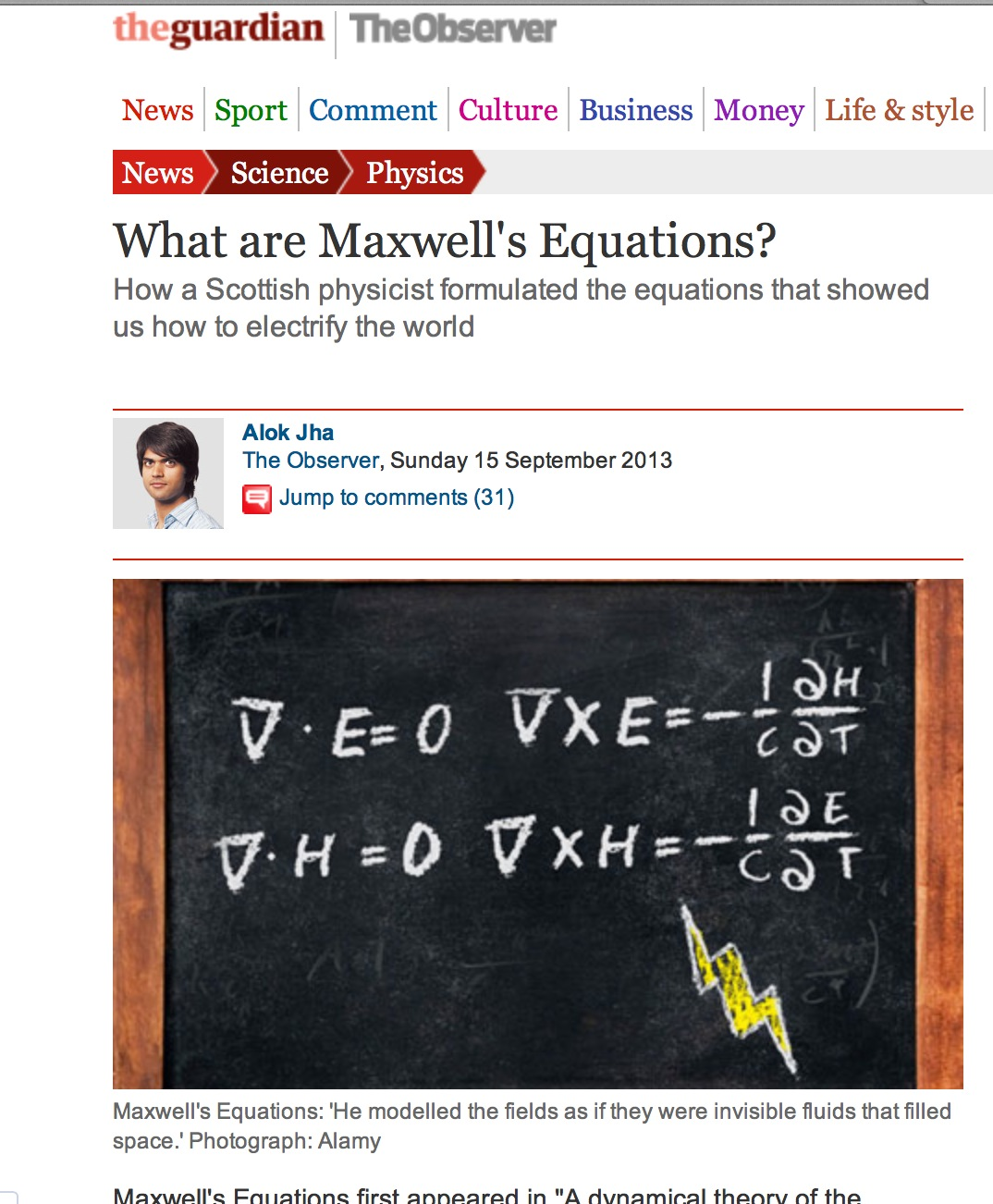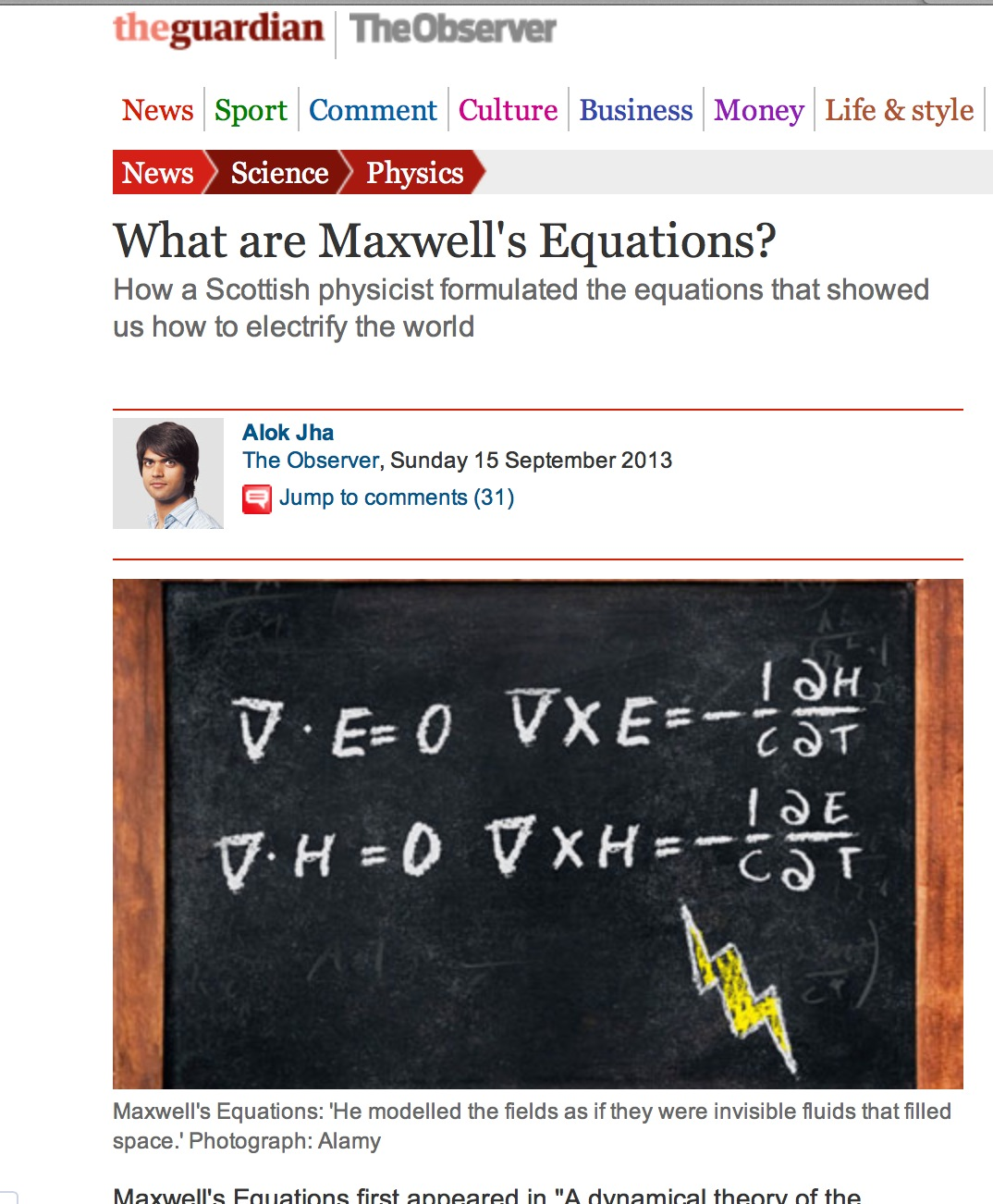### Electromagnetism redefined?

I have finally had some time to catch up with the brand new Observer Tech Monthly magazine, a very welcomed addition to the fine Guardian and Observer newspapers. So, there I was, reading about Paul Mason and his tech, and how the body clock works. So, after a turn of the page I find an article by Alok Jha explaining Maxwell’s Equations and how they electrified the world. All great, except… except… well… except the equations they framed (as expected written with chalk on a blackboard) are incorrect. OK, at least one of them is incorrect , but that it enough to redefine the entire electromagnetic theory.They have started by showing the equations for the case of a region with no charges ($\rho=0$) and no currents ($J=0$), such as in a vacuum. The correct set of Maxwell’s equations reduce in that case to:

•$\nabla \cdot {\bf E}=0$
•$\nabla\cdot {\bf B}=0$
•$\nabla\times {\bf E}=-\frac{\partial {\bf B}}{\partial t}$
•$\nabla\times {\bf B}=\frac{1}{c^2}\frac{\partial {\bf E}}{\partial t}$

I have used the notation${\bf B}$ for the magnetic field… In any case, note the last two equations I wrote above. Can you see the difference between them and the ones depicted in the newspaper article? I wonder what sort of electromagnetic phenomena could be observed by the redefined equations in the Observer… who knows perhaps that is the way electromagnetic fields behave in another Universe, but not on this one.